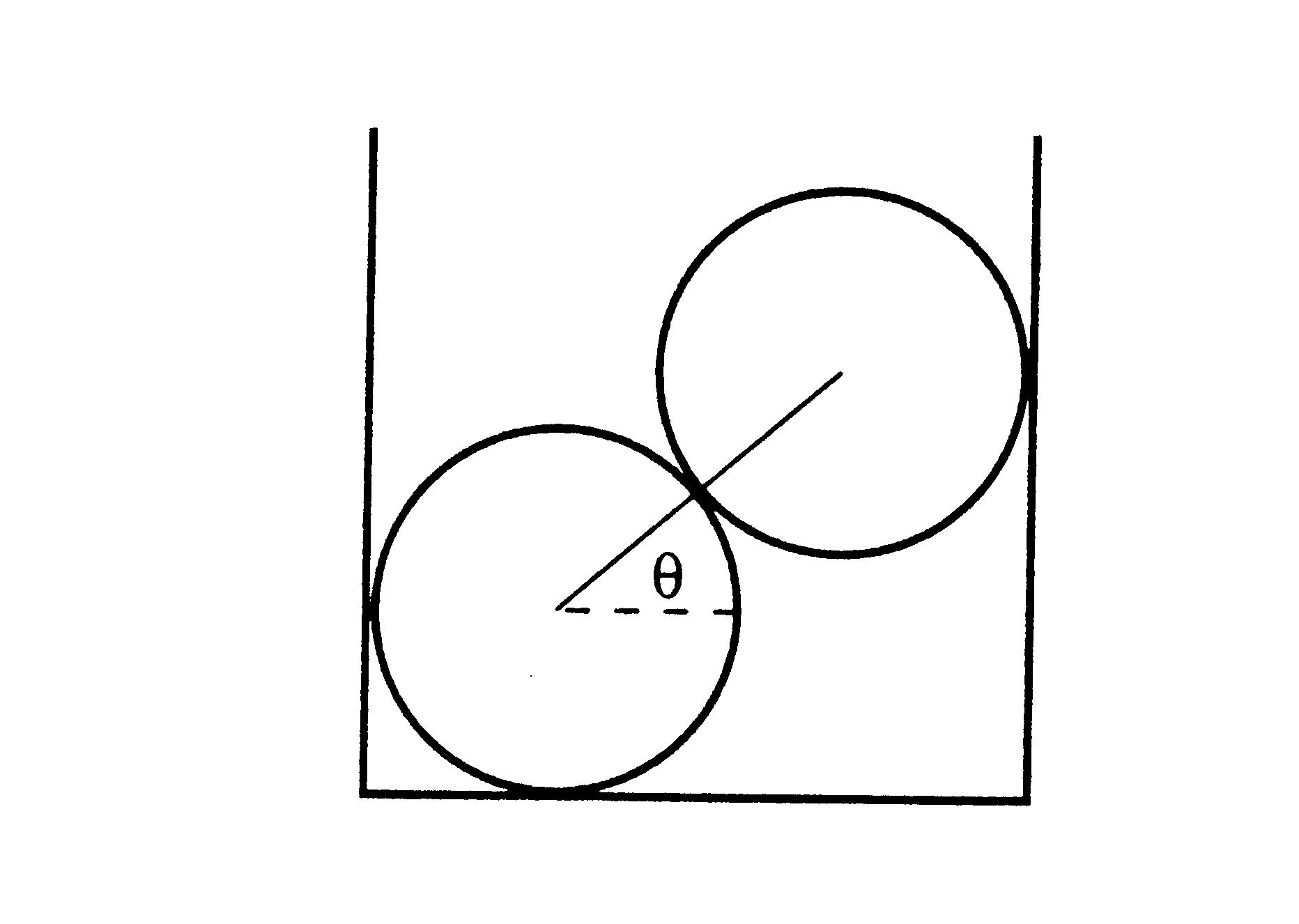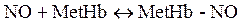Physics 266 Lab I

1) Two logs, each of weight W, lie in a trough with vertical walls in such a way that when viewed end-on the line between their centers makes an angle q with the horizontal. The magnitude of the forces on the bottom log due to the top log, the bottom of the trough, and one wall on the trough are F12, F1b, and F1w, respectively. Similarly the forces on the top log due to the bottom one and the other wall are F21 and F2w. Since F12 = F21, three equations for static equilibrium are

-F12 cos(q) + F1w = 0

F1b - W - F12 sin(q) = 0

F12 cos(q) - F2w = 0

Assuming that all frictional forces are negligible. Find a fourth equation and use Maple to

1. Find the magnitude of the forces F12, F1b, F1w, and F2w. That is find the forces in terms of W and q.
2. Check the solutions2)Consider the binding of nitric oxide to methemoglobinLet K be the dissociation equilibrium constant so that

K = [NO][MetHb]/[MetHb-NO].

Take K to be 250 micromolar, expressing all values in micromolar, solve for how much free NO and MetHb are present when the initial concentrations of NO and MetHb are 30 and 1000 microlmolar.